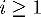Next: Calculating moving averages Up: Pencil and Paper Previous: Forecasting life extension

Calculating feedback models

The thermostat feedback system modeled by the Excel workspace FEEDLAB.XLS (see page) isn't really practical to work by hand. The amount of calculation required to simulate one day is such that you'll almost certainly give up before gaining the intuitive understanding of feedback that comes from changing parameters and seeing the computer immediately simulate the consequences.

Nonetheless, here is the mathematical basis of the model. From these equations you can implement the simulation in another spreadsheet or as a standalone program. The parameters that control the model are identified by their labels on the FEEDLAB control panel.

Given:

 A = Range D = Delay G = Goal L = Insulation N = Noise P = Power R = Random

Calculate the following variables for each time step i:

 Oi = Outside temperature Ei = Error (deviation from goal) Ci = Correction applied by feedback Ti = Inside temperature

with the following initial conditions:

 O0 = 70 T0 = 70

For each 10 minute time intervalcalculate the inside temperature Ti as follows.is a pseudorandom number ranging from -1 to 1, with a new value generated at each occurrence of.By John Walker